Search
Generic selectors
Exact matches only
Search in title
Search in content
Post Type Selectors
Search in posts
Search in pages

# (C) Understanding Learning Tracks and where to start.

You are here:

In the Rocket Math Online Game every student needs to be started at Set A in one of the operations or Learning Tracks. A student’s Learning Track can be changed at any time, but one must be chosen to begin with.  [Exception for students set up through Clever: they all begin with Addition.]  Click here for “How to assign Learning Tracks.”

###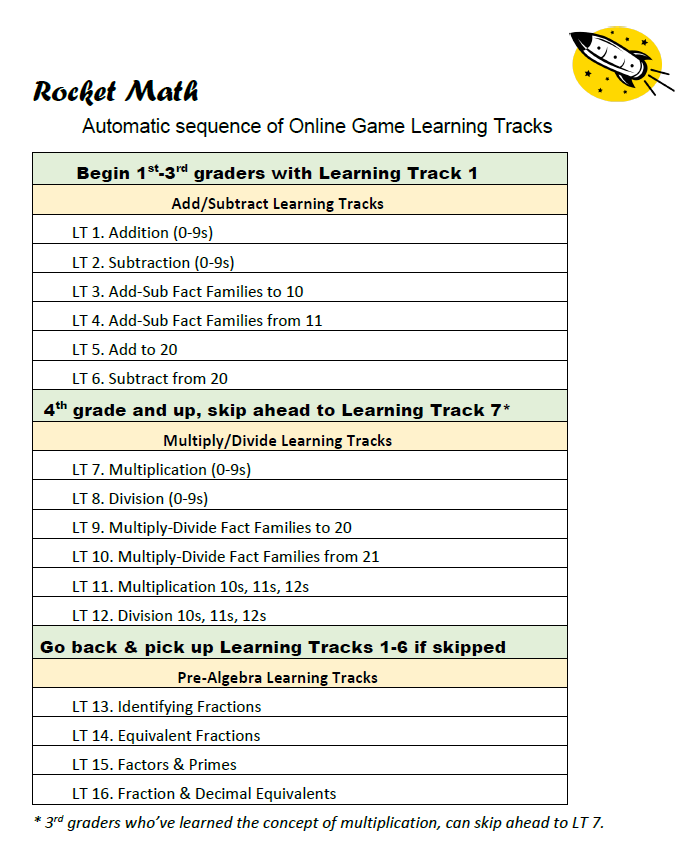Considerations, or what to start with and when?

Note: Compared to the Worksheet Program, the Online Game moves students through the Learning Tracks much faster–in a few weeks, rather than months.  Therefore, it doesn’t hurt to start everyone of the same age in Set A and send them through in the same order. Read about how you can enable Fluency Promotions to move students through a Learning Track they know well in minutes.

### First through 3rd grade students should begin with Learning Track 1. Addition.

The six Add/Subtract Learning Tracks will probably keep your students engaged through first and second grade and into 3rd grade.  However, before any really speedy ones go past Learning Track 6, you should ensure that they understand multiplication.  To know if they understand multiplication ask them to “figure out” the answer to a couple of multiplication facts such as 7 times 8 or 6 times 9.

If they cannot figure out the answer, then teach them the concept before letting them start memorizing multiplication facts.

If they have a process for determining the answer–they understand the operation and are ready to continue this sequence.

• LT 1. Addition (0 – 9s)
• LT 2. Subtraction (0 – 9s)
• LT 3. Add-Subtract Fact Families to 10.
• LT 4. Add-Subtract Fact Families from 11.
• LT 5. Add to 20.
• LT 6. Subtract from 20

### Followed by Multiply/Divide Learning Tracks (once the concept is taught)

• LT 7. Multiplication (0 – 9s)
• LT 8. Division (0 – 9s)
• LT 9. Multiply-Divide Fact Families to 20.
• LT 10. Multiply-Divide Fact Families from 21.
• LT 11. Multiplication 10s-11s-12s
• LT 12. Division 10s-11s-12s

### Grades 4 and up–Start them on LT 7. Multiplication which has priority. *

We are assuming that the concept of multiplication is taught during the first semester of 3rd grade, after which students can begin memorizing multiplication facts. (See above to check on readiness for multiplication facts.)

*Multiplication has priority only because you can’t be sure that you will have a student long enough to teach all four operations.  If students go on to fourth grade somewhere else with only one operation mastered, it better be multiplication.  If they have to, they can keep counting addition and subtraction on their fingers, and they have a chance to survive if they know the multiplication facts.

• LT 7. Multiplication (0 – 9s)
• LT 8. Division (0 – 9s)
• LT 9. Multiply-Divide Fact Families to 20.
• LT 10. Multiply-Divide Fact Families from 21.
• LT 11. Multiplication 10s-11s-12s
• LT 12. Division 10s-11s-12s

### Then go back to Add/Subtract Learning Tracks

Once multiplication and division are mastered, 3rd grade and above can go back to pick up addition and subtraction.

• LT 1. Addition (0 – 9s)
• LT 2. Subtraction (0 – 9s)
• LT 3. Add-Subtract Fact Families to 10.
• LT 4. Add-Subtract Fact Families from 11.
• LT 5. Add to 20.
• LT 6. Subtract from 20

## Then on to our unique Pre-Algebra Learning Tracks.

Identifying Fractions, Equivalent Fractions, Factors & Primes, and Fraction & Decimal Equivalents.   These are most valuable after the basics in grade 3, 4, 5 and up, in this order:

###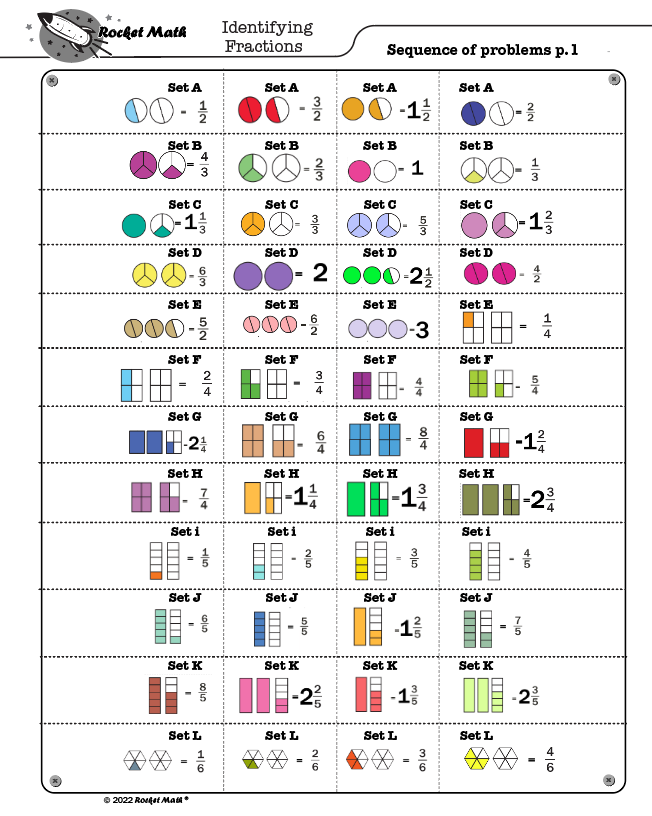LT. 13 Identifying Fractions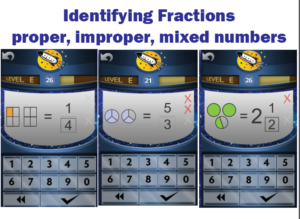Learning to name fractions shown in a picture as parts shaded.   Fractions are always shown with two or more whole units with a number of parts shaded. Students learn from examples of proper fractions, improper fractions such as 5/3 that are equal to more than 1, and mixed numbers such as 2 and 1/2.

### LT. 14 Equivalent Fractions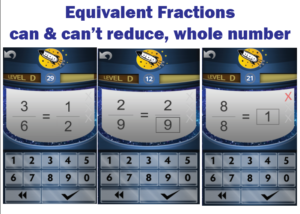Learning by memory the lowest terms of common fractions is very valuable.  Students also learn a lot of fractions that can’t be reduced to lower terms, such as 2/9 =2/9 or 1/4 = 1/4.  They learn to identify many fractions that are equal to one.

### LT. 15 Factors & Primes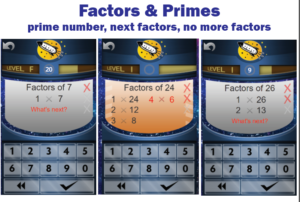Learning all the pairs of factors, by indicating what pair comes next.  Students learn them in order and learn to indicate when there are no more pairs of factors.  Also, learning many common prime numbers and that they only have 1 and themselves as factors.

### LT. 16 Fraction & Decimal Equivalents.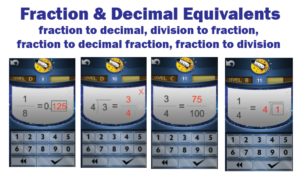Learning common fractions and their decimal equivalents, learning fractions and their fraction equivalents in 10ths or 100ths. Students also learn to change a fraction into a division problem and a division problem into a fraction–something that causes a lot of errors in pre-algebra.